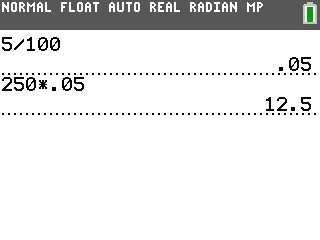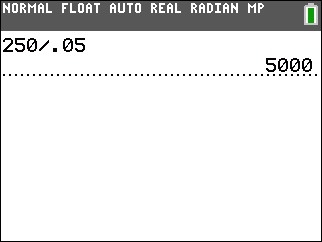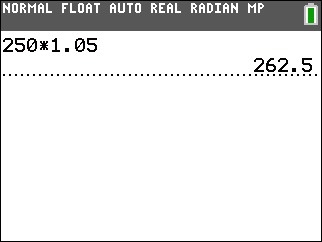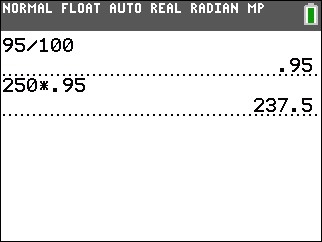# Knowledge Base

## Solution 34812: Percent (%) Operator on the TI-83 Plus and TI-84 Plus Family.

### Does the TI-83 Plus and TI-84 Plus Family use the percent operator (%)?

The TI-83 Plus and TI-84 Plus Family does not include a percent operator (%). Therefore, in order to convert a number to a percentage, simply divide that number by 100. Likewise, to convert a percentage to a number, multiply that number by 100. The following examples demonstrate exactly how to perform some percentage-related calculations:

Example - Percentage: Calculate 5% of 250.

1) Press  [÷]    [ENTER] to find the percentage in decimal format.
2) Press    [×] [.]   [ENTER].Example - Ratio: Calculate the ratio of 250 to 5.

1) Press    [÷] [.]   [ENTER].